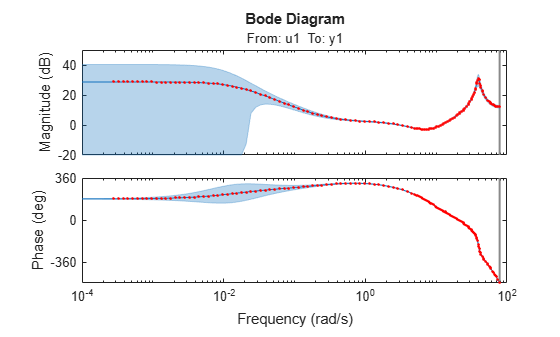Documentation

### This is machine translation

Mouseover text to see original. Click the button below to return to the English version of the page.

# canon

State-space canonical realization

## Syntax

```csys = canon(sys,type) [csys,T]= canon(sys,type) csys = canon(sys,'modal',condt) ```

## Description

`csys = canon(sys,type)` transforms the linear model `sys` into a canonical state-space model `csys`. The argument `type` specifies whether `csys` is in modal or companion form.

```[csys,T]= canon(sys,type)``` also returns the state-coordinate transformation `T` that relates the states of the state-space model `sys` to the states of `csys`.

`csys = canon(sys,'modal',condt)` specifies an upper bound `condt` on the condition number of the block-diagonalizing transformation.

## Input Arguments

 `sys` Any linear dynamic system model, except for `frd` models. `type` Canonical form of `csys`, specified as one of the following values: `'modal'` — convert `sys` to modal form.`'companion'` — convert `sys` to companion form. `condt` Positive scalar value specifying an upper bound on the condition number of the block-diagonalizing transformation that converts `sys` to `csys`. This argument is available only when `type` is `'modal'`. Increase `condt` to reduce the size of the eigenvalue clusters in the A matrix of `csys`. Setting `condt = Inf` diagonalizes A. Default: `1e8`

## Output Arguments

 `csys` State-space (`ss`) model. `csys` is a state-space realization of `sys` in the canonical form specified by `type`. `T` Matrix specifying the transformation between the state vector x of the state-space model `sys` and the state vector xc of `csys`:xc = Tx. This argument is available only when `sys` is state-space model.

## Examples

collapse all

Consider a system with doubled poles and clusters of close poles:

`$G\left(s\right)=100\frac{\left(s-1\right)\left(s+1\right)}{s\left(s+10\right)\left(s+10.0001\right){\left(s-\left(1+i\right)\right)}^{2}{\left(s-\left(1-i\right)\right)}^{2}}$`

Create a linear model of this system, and convert it to modal canonical form.

```G = zpk([1 -1],[0 -10 -10.0001 1+1i 1-1i 1+1i 1-1i],100); Gc = canon(G,'modal');```

The system, `G`, has a pair of nearby poles at $s=-10$ and $s=-10.0001$. `G` also has two complex poles of multiplicity 2 at $s=1+i$ and $s=1-i$. As a result, the modal form has a block of size 2 for the two poles near $s=-10$, and a block of size 4 for the complex eigenvalues.

`Gc.A`
```ans = 7×7 0 0 0 0 0 0 0 0 1.0000 1.0000 0 0 0 0 0 -1.0000 1.0000 2.0548 0 0 0 0 0 0 1.0000 1.0000 0 0 0 0 0 -1.0000 1.0000 0 0 0 0 0 0 0 -10.0000 8.0573 0 0 0 0 0 0 -10.0001 ```

Separate the two poles near $s=-10$ by increasing the value of the condition number of the block-diagonalizing transformation. The default value of the condition number is 1e8.

```Gc2 = canon(G,'modal',1e10); Gc2.A```
```ans = 7×7 0 0 0 0 0 0 0 0 1.0000 1.0000 0 0 0 0 0 -1.0000 1.0000 2.0548 0 0 0 0 0 0 1.0000 1.0000 0 0 0 0 0 -1.0000 1.0000 0 0 0 0 0 0 0 -10.0000 0 0 0 0 0 0 0 -10.0001 ```

The `A` matrix of `Gc2` includes separate diagonal elements for the poles near $s=-10$. The cost of increasing the condition number of `A` is that the `B` matrix includes some large values.

```format shortE Gc2.B```
```ans = 7×1 3.2000e-01 -6.5691e-03 5.4046e-02 -1.9502e-01 1.0637e+00 3.2533e+05 3.2533e+05 ```

Estimate a state-space model that is freely parameterized.

```load icEngine.mat z = iddata(y,u,0.04); FreeModel = n4sid(z,4,'InputDelay',2);```

Convert the estimated model to companion canonical form.

`CanonicalModel = canon(FreeModel,'companion');`

Obtain the covariance of the resulting form by running a zero-iteration update to model parameters.

```opt = ssestOptions; opt.SearchOptions.MaxIterations = 0; CanonicalModel = ssest(z,CanonicalModel,opt);```

Compare frequency response confidence bounds of `FreeModel` to `CanonicalModel`.

```h = bodeplot(FreeModel,CanonicalModel,'r.'); showConfidence(h)```The frequency response confidence bounds are identical.

collapse all

### Modal Form

In modal form, A is a block-diagonal matrix. The block size is typically 1-by-1 for real eigenvalues and 2-by-2 for complex eigenvalues. However, if there are repeated eigenvalues or clusters of nearby eigenvalues, the block size can be larger.

For example, for a system with eigenvalues $\left({\lambda }_{1},\sigma ±j\omega ,{\lambda }_{2}\right)$, the modal A matrix is of the form

`$\left[\begin{array}{cccc}{\lambda }_{1}& 0& 0& 0\\ 0& \sigma & \omega & 0\\ 0& -\omega & \sigma & 0\\ 0& 0& 0& {\lambda }_{2}\end{array}\right]$`

### Companion Form

In the companion realization, the characteristic polynomial of the system appears explicitly in the rightmost column of the A matrix. For a system with characteristic polynomial

`$p\left(s\right)={s}^{n}+{\alpha }_{1}{s}^{n-1}+\dots +{\alpha }_{n-1}s+{\alpha }_{n}$`

the corresponding companion A matrix is

`$A=\left[\begin{array}{cccccc}0& 0& ..& ..& 0& -{\alpha }_{n}\\ 1& 0& 0& ..& 0& -{\alpha }_{n}-1\\ 0& 1& 0& .& :& :\\ :& 0& .& .& :& :\\ 0& .& .& 1& 0& -{\alpha }_{2}\\ 0& ..& ..& 0& 1& -{\alpha }_{1}\end{array}\right]$`

The companion transformation requires that the system be controllable from the first input. The companion form is poorly conditioned for most state-space computations; avoid using it when possible.

## Algorithms

The `canon` command uses the `bdschur` command to convert `sys` into modal form and to compute the transformation `T`. If `sys` is not a state-space model, the algorithm first converts it to state space using `ss`.

The reduction to companion form uses a state similarity transformation based on the controllability matrix .

## References

 Kailath, T. Linear Systems, Prentice-Hall, 1980.